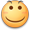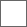### 其实看了看氯化钠的普攻倍率感觉不算低了80+80+100+100+250=61080+80+100+100+250=610分支一套计算下来伤害其实也不低
氯化钠 3*30+4*30+80+4*60+250(空间核心) 780*3+1000 （满10S律者）
强 210*6+260=1520(6下)
鹦 180+280=460
绯 7*60*2=840(sss)

但是相比下氯化钠的律着模式太难以进入 而其他角色的分支很容易打出 而氯化钠的普通攻击加伤几乎没有你这普攻一套下来几秒？别的靠普攻输出的几秒？#### 扫二维码下载贴吧客户端

下载贴吧APP
看高清直播、视频！